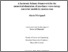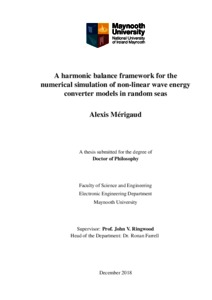# A harmonic balance framework for the numerical simulation of non-linear wave energy converter models in random seas

Mérigaud, Alexis (2018) A harmonic balance framework for the numerical simulation of non-linear wave energy converter models in random seas. PhD thesis, National University of Ireland Maynooth.Previewmore...Add this article to your Mendeley library

## Abstract

Numerical simulation is essential, to assist in the development of wave energy technology. In particular, tasks such as power assessment, optimisation and structural design require a large number of numerical simulations to calculate the wave energy converter (WEC) outputs of interest, over a variety of wave conditions or physical parameters. Such challenges involve a sound understanding of the statistical properties of ocean waves, which constitute the forcing inputs to the wave energy device, and computationally efficient numerical techniques for the speedy calculation of WEC outputs. This thesis studies the statistical characterisation, and numerical generation, of ocean waves, and proposes a novel technique for the numerical simulation of non-linear WEC models. The theoretical foundations, the range of validity, and the importance of the statistical representation of ocean waves are first examined. Under relatively mild assumptions, ocean waves can be best described as a stationary Gaussian process, which is entirely characterised by its spectral density function (SDF). Various wave superposition techniques are discussed and rigorously compared, for the numerical generation of Gaussian wave elevation time series from a given SDF. In particular, the harmonic random amplitude (HRA) approach can simulate the target statistical properties with perfect realism. In contrast, the harmonic deterministic amplitude (HDA) approach is statistically inconsistent (because the generated time-series are non-Gaussian, and under-represent the short-term statistical variability of real ocean waves), but can be advantageous in the context of WEC simulations since, if it can be verified that HDA results are unbiased, the HDA method requires a smaller number of random realisations than the HRA method, to obtain accurate WEC power estimates. When either HDA or HRA are used for the generation of wave inputs, the forcing terms of WEC mathematical models are periodic. Relying on a Fourier representation of the system inputs and variables, the harmonic balance (HB) method, which is a special case of spectral methods, is a suitable mathematical technique to numerically calculate the steady-state response of a non-linear system, under a periodic input. The applicability of the method to WEC simulation is demonstrated for those WEC models which are described by means of a non-linear integro-differential equation. In the proposed simulation framework, the WEC output, in a given sea state, is assessed by means of many, relatively short, simulations, each of which is efficiently solved using the HB method. A range of four case studies is considered, comprising a flap-type WEC, a spherical heaving point-absorber, an array of four cylindrical heaving point-absorbers, and a pitching device. For each case, it is shown how the HB settings (simulation duration and cut-off frequency) can be calibrated. The accuracy of the HB method is assessed through a comparison with a second-order Runge-Kutta (RK2) time-domain integration scheme, with various time steps. RK2 results converge to the HB solution, as the RK2 time step tends to zero. Furthermore, in a Matlab implementation, the HB method is between one and three orders of magnitude faster than the RK2 method, depending on the RK2 time step, and on the method chosen for the calculation of the radiation memory terms in RK2 simulations. The HB formalism also provides an interesting framework, for studying the sensitivity of the WEC dynamics to system parameter variations, which can be utilised within a gradient-based parametric optimisation algorithm. An example of WEC gradientbased parametric optimisation, carried out within the HB framework, is provided.

Item Type: Thesis (PhD) harmonic balance framework; numerical simulation; non-linear wave energy converter models; random seas; Faculty of Science and Engineering > Electronic Engineering 10861 IR eTheses 10 Jun 2019 13:32Item control page